Function Repository Resource:

# NearestKroneckerProductSum

Approximate a numerical matrix as sum of Kronecker products

Contributed by: Jan Mangaldan
 ResourceFunction["NearestKroneckerProductSum"][m,{p1,q1},{p2,q2},n] gives a decomposition of a numerical matrix m into a sum of n Kronecker products of matrices of dimensions p1×q1 and p2×q2. ResourceFunction["NearestKroneckerProductSum"][m,{p1,q1},{p2,q2},UpTo[n]] gives the decomposition for n Kronecker products, or as many as are available.

## Details

ResourceFunction["NearestKroneckerProductSum"] returns a list of pairs of matrices {{u1,v1},{u2,v2},}, each of dimensions p1×q1 and p2×q2, such that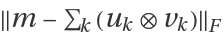is minimized, where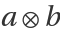is KroneckerProduct[a,b] and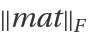is Norm[mat,"Frobenius"].
The lists {p1,q1} and {p2,q2} must be commensurate, and satisfy Dimensions[m]={p1p2,q1q2}.
ResourceFunction["NearestKroneckerProductSum"] takes the same options as SingularValueDecomposition.

## Examples

### Basic Examples (2)

Compute a Kronecker product approximation of a small matrix:

 In:=Out=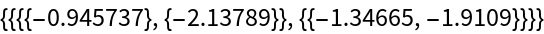Form the approximant to the original matrix:

 In:=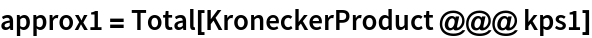Out=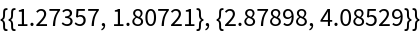Using two terms gives a much better approximant:

 In:=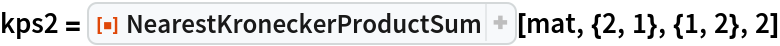Out=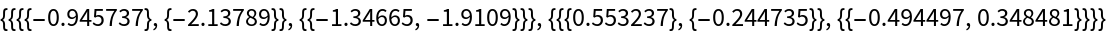In:=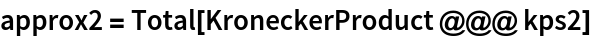Out=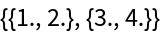The Frobenius norm of the remainder matrix is on the order of machine precision:

 In:=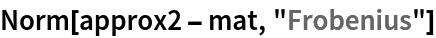Out=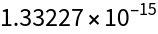Define a 10×12 matrix:

 In:=Express the matrix as a sum of three Kronecker products of matrices of dimensions 5×3 and 2×4:

 In:=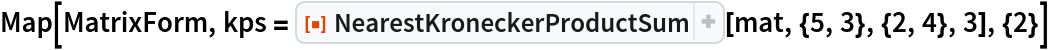Out=Check the difference between the original matrix and the sum of the Kronecker products:

 In:=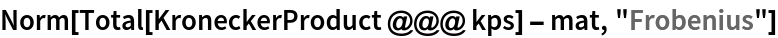Out=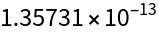### Scope (3)

A 10×12 matrix with approximate numerical values:

 In:=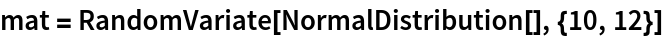Out=Express the matrix as a sum of up to seven Kronecker products of matrices of dimensions 2×3 and 5×4:

 In:=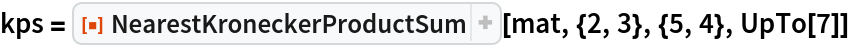Out=Check the difference between the original matrix and the sum of the Kronecker products:

 In:=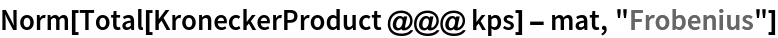Out=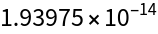An arbitrary-precision 10×12 matrix:

 In:=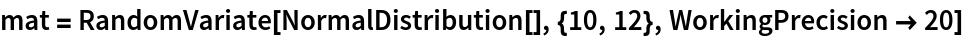Out=Express the matrix as a sum of up to seven Kronecker products of matrices of dimensions 2×3 and 5×4:

 In:=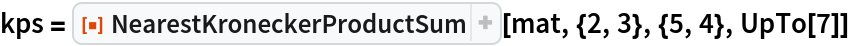Out=Check the difference between the original matrix and the sum of the Kronecker products:

 In:=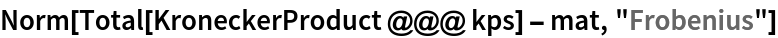Out=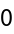Decompose a random complex-valued 10×12 matrix into a sum of two Kronecker products of matrices of dimensions 2×3 and 5×4:

 In:=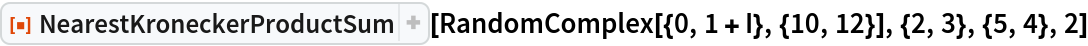Out=### Possible Issues (4)

NearestKroneckerProductSum is left unevaluated for symbolic input:

 In:=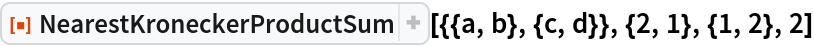Out=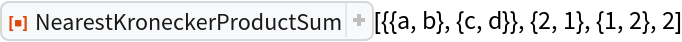NearestKroneckerProductSum is left unevaluated for exact input:

 In:=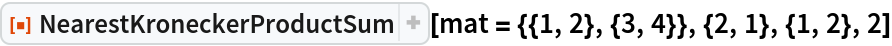Out=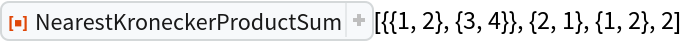Numericize the input to get a result:

 In:=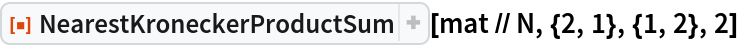Out=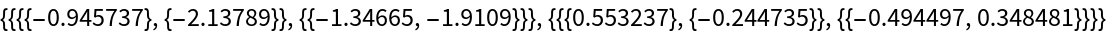NearestKroneckerProductSum is left unevaluated if the specified dimensions are incommensurate:

 In:=Out=The decomposition of a matrix into a sum of Kronecker products is not unique:

 In:=In:=Out=## Version History

• 1.0.0 – 25 January 2021